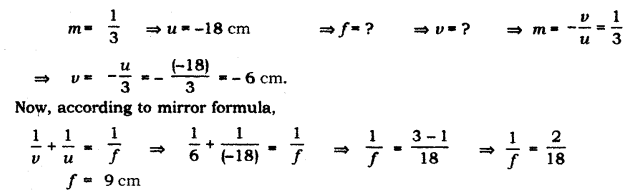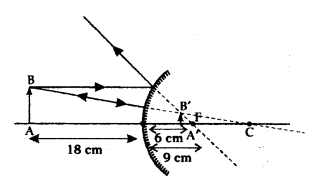# List the new Cartesian sign convention for reflection of light by spherical mirror

List the new Cartesian sign convention for reflection of light by spherical mirror. Draw a diagram and apply these conventions for calculating the focal length and nature of spherical mirror which forms a 1/3 times magnified virtual image of an object placed 18 cm in front of it

New Cartesian Sign Conventions for reflection of light by spherical mirrors are given
below:

1. The object is always placed to the left of the mirror. This implies that the light from the object falls on the mirror from the left-hand side.
2. All distances parallel to the principal axis are measured from the pole of the mirror.
3. All the distances measured to the right of the origin (along + x-axis) are taken as positive while those measured to the left of the origin (along - x-axis) are taken as negative.
4. Distances measured perpendicular to and above the principal axis (along + y-axis) are taken as positive.
5. Distances measured perpendicular to and below the principal axis (along - y-axis) are taken as negative.Since, the focal length is positive so, it is a convex mirror.
Ray diagram: